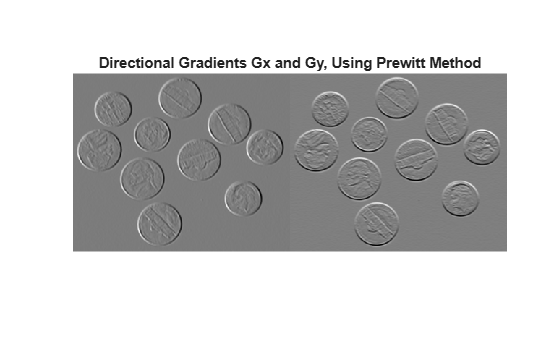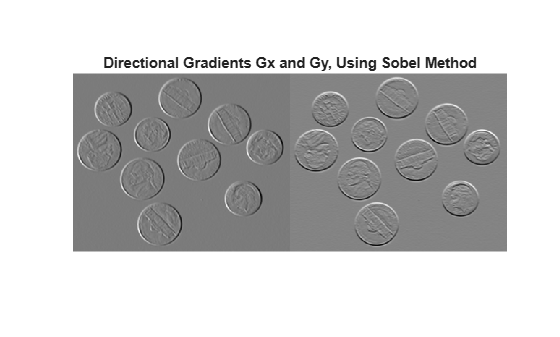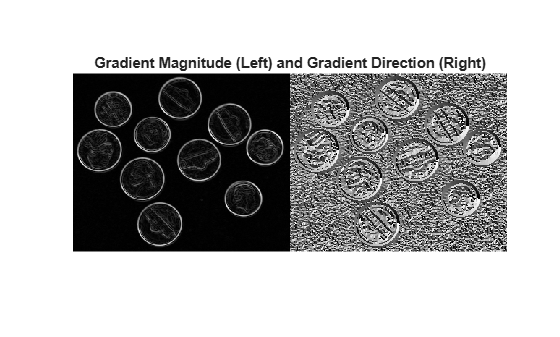Find directional gradients of 2-D image

## Syntax

``````[Gx,Gy] = imgradientxy(I)``````
``````[Gx,Gy] = imgradientxy(I,method)``````

## Description

example

``````[Gx,Gy] = imgradientxy(I)``` returns the directional gradients, `Gx` and `Gy` of the grayscale or binary image `I`.You optionally can compute the directional gradients using a GPU (requires Parallel Computing Toolbox™).```

example

``````[Gx,Gy] = imgradientxy(I,method)``` returns the directional gradients using the specified `method`.```

## Examples

collapse all

`I = imread('coins.png');`

`[Gx, Gy] = imgradientxy(I,'prewitt');`

```figure imshowpair(Gx, Gy, 'montage'); title('Directional Gradients: x-direction, Gx (left), y-direction, Gy (right), using Prewitt method')````I = imread('coins.png');`

Calculate the x- and y-directional gradients. By default, `imgradientxy` uses the Sobel gradient operator.

`[Gx,Gy] = imgradientxy(I);`

```imshowpair(Gx,Gy,'montage') title('Directional Gradients Gx and Gy, Using Sobel Method')````[Gmag,Gdir] = imgradient(Gx,Gy);`

Display the gradient magnitude and direction.

```imshowpair(Gmag,Gdir,'montage') title('Gradient Magnitude (Left) and Gradient Direction (Right)')```## Input Arguments

collapse all

Input image, specified as a 2-D grayscale image or 2-D binary image.

Data Types: `single` | `double` | `int8` | `int32` | `uint8` | `uint16` | `uint32` | `logical`

Gradient operator, specified as one of the following values.

MethodDescription
`'sobel'`

Sobel gradient operator. The gradient of a pixel is a weighted sum of pixels in the 3-by-3 neighborhood. In the vertical (y) direction, the weights are:

```[ 1 2 1 0 0 0 -1 -2 -1 ]```
In the x direction, the weights are transposed.

`'prewitt'`

Prewitt gradient operator. The gradient of a pixel is a weighted sum of pixels in the 3-by-3 neighborhood. In the vertical (y) direction, the weights are:

```[ 1 1 1 0 0 0 -1 -1 -1 ]```
In the x direction, the weights are transposed.

`'central'`

Central difference gradient. The gradient of a pixel is a weighted difference of neighboring pixels. In the y direction, ```dI/dy = (I(y+1) - I(y-1))/2```.

`'intermediate'`

Intermediate difference gradient. The gradient of a pixel is the difference between an adjacent pixel and the current pixel. In the y direction, ```dI/dy = I(y+1) - I(y)```.

Data Types: `char` | `string`

## Output Arguments

collapse all

Horizontal gradient, returned as a numeric matrix of the same size as image `I`. The horizontal (x) axis points in the direction of increasing column subscripts. `Gx` is of class `double`, unless the input image `I` is of class `single`, in which case `Gx` is of class `single`.

Data Types: `single` | `double`

Vertical gradient, returned as a numeric matrix of the same size as image `I`. The vertical (y) axis points in the direction of increasing row subscripts. `Gy` is of class `double`, unless the input image `I` is of class `single`, in which case `Gy` is of class `single`.

Data Types: `single` | `double`

## Tips

• When applying the gradient operator at the boundaries of the image, values outside the bounds of the image are assumed to equal the nearest image border value.

## Algorithms

The algorithmic approach is to compute directional gradients with respect to the x-axis and y-axis. The x-axis is defined along the columns going right and the y-axis is defined along the rows going down.

`imgradientxy` does not normalize the gradient output. If the range of the gradient output image has to match the range of the input image, consider normalizing the gradient image, depending on the `method` argument used. For example, with a Sobel kernel, the normalization factor is 1/8, and for Prewitt, it is 1/6.